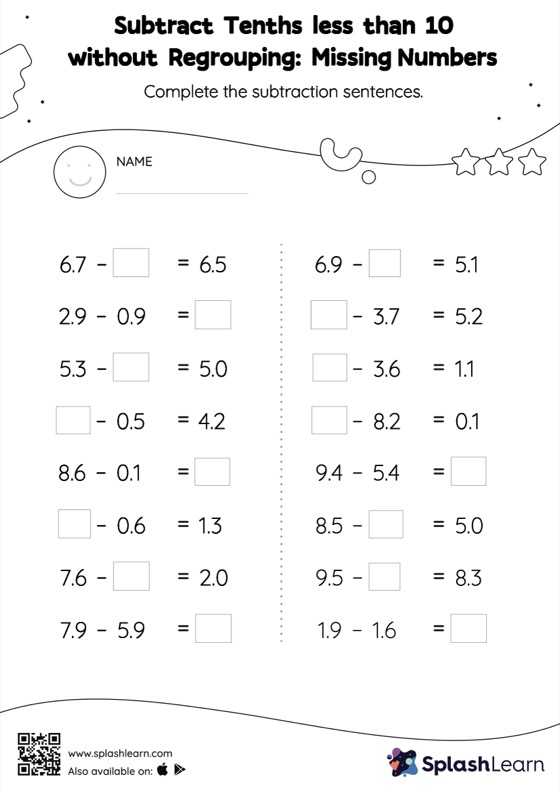# Subtract Tenths less than 10 without Regrouping: Missing Numbers Worksheet

Home > Subtract Tenths less than 10 without Regrouping: Missing NumbersStudents find the missing number while subtracting decimals by using the relationship between addition and subtraction. Subtract tenths less than 10 without regrouping worksheet does not require them to regroup numbers. This worksheet is about practicing with the horizontal format in which numbers are written side by side. To develop flexibility with numbers and operations, students need to have enough practice in this format and not just rely on the vertical/column method.